# Physics - Electromagnetism - Explaining the Physics behind Electromotors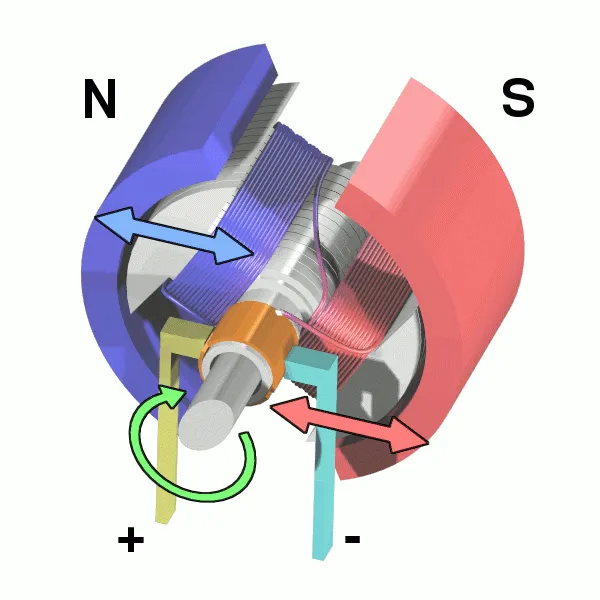Image source:## Introduction

Hello it's a me again Drifter Programming! Today we continue with Electromagnetism to get into how electromotors work. We already did a lot of the Physics needed in the previous two posts that I suggest you reading, but don't worry a lot will be explained again to make the explanation more understandable. So, without further do, let's get started!

## Nikola Tesla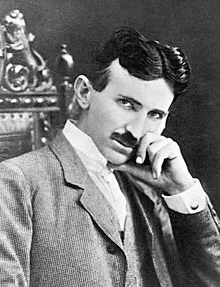Image source: https://en.wikipedia.org/wiki/Nikola_Tesla

Before getting into the explanation we should first get into some History. Without the work of Nikola Tesla, a Serbian Inventor that studied Alternating Current (AC), electric motors would not be that feasible and efficient as they are now. You might know Elon Musk's Electric Car Company "TESLA" that constructs electric cars. Such vehicles would be impossible without the work of Nikola Tesla. In his age a lot of Scientists and Engineers believed that a motor could not be operated without bruches and commutators. There just couldn't be a motor without a mechanical mean of some kind that generates the current. Tesla tried to solve this problem and also got to a solution.

Tesla worked out the theory and also tested and confirmed all his theoretical conclusions with experiments. In his lab he produced several types of practical motors. Note that he got to all this conclusions without having anything similar to learn from in the whole scientific literature, cause no-one at his time believed that such things where possible. Most patents in his time didn't even have a reasonable reference design and explanation and the inventions where questionable. Prof. Ferraris essay anticipated the date of his application even if the application of Prof. Ferraris was six or seven months after the date of filling out Tesla's patents. Prof. Ferraris stated that the transmission power could be usable only when having a property constructed generator and that the required difference of phase without losses, even with condensers, could not be obtained. Many electricians in England even called Tesla an Imitator and stated that he stole the work from Ferraris! But, as we all know his inventions where original work and he was a great Inventor. So sad that he never got appreciated about it when he was still alive...

Some of the generators that Tesla invented where generators with two or three circuits of differing phase, a three-wire system, the closed coil armature, motors with direct current in the field. The most practicable form of an electromotors was the two-wire motor that contained a single energizing circuit and induced circuits. We will later on explain how the simplest form of motor works!

All this information is from Nikola Tesla's paper released in 1891.

## The History of Electromotors

The history is long and contains many different inventions and scientists. To stay organized let's make an unordered-list with the most important points.

### Early motors:

•  The first electric motors were simple electrostatic devices described by Andrew Gordon and Benjamin Franklin in the 1740s.
• In 1820 Andre-Marie Ampere described the theorical principle behind them in his law that is now known as Ampere's force law. The law describes the mechanical force by the interactions of an electric current and a magnetic field.
• The conversion of the electrical energy into mechanical energy by electromagnetic was demonstrated by Michael Faraday in 1821. He dipped a free-hanging wire into a pool of mercury on which a permanent magnet was placed. When current passed through the wire then the wire rotated around the magnet which showed that the current gave rise to a closed circular magnetic field around the wire.
• Barlow's wheel was a refinement of Faraday's demonstration and this and the similar homopolar motors remained unsuited in applications until late in the century.
• In 1827 Anyos Jedlik experimented with electromagnetic coils. He solved the the theoretical problems of continuous rotation by inventing the commutator.
• In 1828 Jedlik demonstrated the first device that contained the three main component of practical DC motors: the stator, rotor and commutator. This device contained no permanent magnets and the magnetic field were produced by the currents flowing through their windings.

### DC motors:

• The first commutator DC electric motor that was capable of turning machinery was invented by William Sturgeon in 1832. In 1837 Thomas Davenport patented his design. The primary battery power cost was very high and so these motors were unsuccessful. Most inventors following them encountered the same battery cost issues.
• As we saw till now most attempts till then where relatively weak rotating motors that needed a lot of battery power. Moritz von Jacobi created the first real rotating electric motor in 1834. This device developed a remarkable mechanical output power. He even improved his design in 1838. In 1839/40 and afterwards developers managed to build similar and higher performance motors.
• In 1855 Jedlik built a device similar to electromagnetic self-rotors that was capable of useful work and was even used in building a model of an electric vehicle.
• Antonio Pacinotti in 1864 described the first ring armature which was a major turning point. He featured symmetrically-grouped coils closed upon themselves and connected to the bars/brushes of a commutator.
• In 1871 Zenobe Gramme reinventd Pacinotti's design. In 1873 he even showed that his dynamo could also be used as a motor.
• In 1886 Frank Julian Spraque invented the first practical DC motor, which was an non-sparking device that maintened relatively constant speed under variable loads. His inventions improved grid electric distribution. In 1887-88 Spraque invented the first electric trolley system in Richmond Virginia and provided control systems for the electric operations. In 1892 he also created the first electric elevator and control system and even an electric subway. His inventions made a great impact in the industry for the use of electromotors. Electric motors revolutionized industry. Industrial processes were no longer limited by power transmission and every machine could be equipped with its own power source, providing easy control at the point of use.

### AC motors:

• In 1824 Francois Arago formulated the existence of magnetic fields. Walter Baily demonstrated the first primitive induction motor in 1879! (in the gap only DC motors were invented) In the 1880's many inventors tried to developed AC motors, because they had advantages in long-distance high-voltage transmission.
• As we already explained before the first alternating-current commutator-less induction motors were  independently invented by Galileo Ferraris and Nikola Tesla in 1885 and 1887.
• In 1888 Tesla presented a paper that described three patented two-phase and four-stator-pole motor types, which were: a reluctance motor, induction motor and synchronous motor.
• One of the patents Tesla filed in 1887. George Westinghouse wanted to employee Tesla to develope his patents and also assigned C. F. Scott to help Tesla, but Tesla left for other pursuits in 1889. Westinghouse successfully adapted the constant speed AC induction power for mining operations in Colorado, in 1891. Using such a motor for street cars was not yet suitable.
• In 1889 and 1890 respectively Mikhail Dolivo-Dobrovolsky invented the three-phase cage-rotor induction motor and three-limb transformer. He also claimed that Tesla's motor was not practical because of two-phase pulsations.
• Westinghouse achieved its first practical induction motor in 1892 and developed a line of polyphase 60 hertz induction motors in 1893. These early Westinghouse motors were two-phase motors with wound rotors
• The General Electric Company began developing three-phase induction motors in 1891. By 1896 General Electric and Westinghouse signed a cross-licensing agreement for the bar-winding-rotor design.

## Explaining the Physics behind Electromotors

We saw many different inventions that are electromotors and how useful they are, but what is the actual basic principle of an electromotor? Motors are the most common application of magnetic force on current-carrying wires. They have loops of wire inside of an magnetic field. As we said last time the magnetic fields exerts torque on those current-carrying loops, which cause the shaft to rotate. The electrical energy is that way converted into mechanical work.

### Basic Physics (that we covered in my series)

To understand the actual effect of the magnetic field to a current-carrying wire we have to understand what happens with single particles. Charged particles that moves inside of a magnetic field are affected by that field. The force exerted on a charged particle is proportional to the magnitude of the charge, the (drifting) velocity of that particle and the magnetic field strength (or magnetic flux density). This means that the force exerted on charged particles is given by: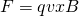You can clearly see that this force is the cross product between the vectors of the velocity and magnetic field. This means that the force is always perpendicular to the motion and so only rotates the particle around an axis. There is no actual kinetic energy loss or gain when moving through an magnetic field. The only things that changes is the direction of movement. The particle is not affected by the field when it moves parallel to the field (φ = 0) and is affected at "max" when moving perpendicular to the field (φ = 90). This all means that the actual force depends on the angle φ between the velocity vector and magnetic field which means that:Current is build up of many moving charges and describes the amount of charges that move through an area in a time unit. Supposing a rectangle loop with lengths a and b with a being on top/botto. The actual force applied on current can be proven to be: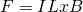We see that the force is again perpendicular to the trajectory L (similar to velocity). The actual force is proportional to the current, length of the wire and magnetic field. The forces on the opposing sides cancel each other out, cause they have the same magnitude of current, but with opposing direction. But, there still is a torque. The total torque is: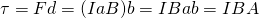where A = ab is the Area of the loop, which of course can be anything.

The magnetic force direciton in charges and current can be found by a corresponding right-hand rule...

The product IA is denoted as μ and called magnetic moment. Magnetic moment can be described for any loop of any shape and size. Because only the perpendicular component is being affected by the magnetic field we of course also have to include the angle θ of μ and B which gives us:Magnetic moment is actually μ=NIA and so the torque in one loop can be multiplied by N which gives us the total torque for N-loops: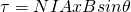The Work produced when moving from an angle φ1 to an angle φ2 is: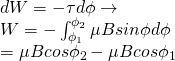with a special case being: W = 2μB when moving from 0 to π (aligned and anti-aligned states). The potential energy difference when W = 2μB is also ΔU = 2μB

Note that we can also get into forces caused on induced voltage, but I don't got into induction in general in my series yet. Either way the magnetic force would then be equal to the emf and so: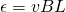Induction is used in induction motors that we will also cover later on...

### Electric motors

Electric motors in general involve rotating coils of wire which are driven by the magnetic force exerted by a magnetic field on electric current. They transform electrical energy into mechanical energy and are of two types:

1. DC motor -> with a direct current battery
2. AC motor -> with alternating current (also called Induction motors)

Let's get into each category on it's own...

### The DC motor

So, how does the basic motor use all this? The magnetic field of course rotates the shaft. Once the loop's surface alignes with the magnetic field the current is reversed so that the torque is continuous. If the current was not being reversed then the torque would make the loop "go back" and start oscillating. The reversal of the current is being done with commutators and brushes. The commutator reverses the current at set points to keep the motor in a continuous motion. The basic commuator has three contact areas to avoid, which are the spots where we would else have zero torque. The brushes press against the commutator and help creating electrical contact between the parts.

You can clearly see that DC motors use the exact principle that we covered in the theory before and so follow the same exact equations and theorems that we analyzed till now...

The following animation shows the forces and motion: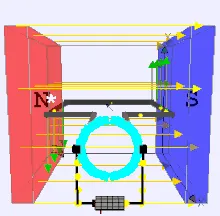Image source: https://en.wikipedia.org/wiki/DC_motor

The current flowing in the parts that are in the front and back is of course parallel to the magnetic field that goes from N to S which clearly shows us that only the other sides are affected by the field.

We can see that the circuit is connected to an energy source (battery), which of course has to be of a special kind so that the current changes direction at the "critical" points. Such an energy source is of course the commutator. Where the rotating coil meets the wires attached to the power source, we find a split ring commutator. The coil turns, but the commutator and power source do not. As the coil turns, it moves off of the box connector and as it continues to turn, it connects to the other box connector (the black boxes that you can see in the animation). As the coil turns, it reverses its connections to the external circuit and therefore the current inside of the coil moves in the same direction, but the current of the actual loop can be reversed. This so called armature (or coil) can that way continue to spin in the same direction all the time. Electric motors consist of many such coils/armatures to provide more uniform torque.

From all that we can see that DC motors are build up of:

• An Armature or rotor
• A commutator
• Brushes
• An Axle
• Permanent magnets
• DC power supply of some sort

The armature (or rotor) of an motor is an electromagnet and the field magnet is a permanent magnet (might also be an electromagnet tho). The Axle holds the armature and commutator. We explained what the commutator and Brushes do...

The same concept can also be used in Electric generators, were the rotation of produces different directions of motion at different points on the circle and so generates a sinusoidal (AC) voltage.

### The AC motor

Because Alternating current (AC) is easier to be supplied (then DC current) in homes, factories and most buildings and little DC batteries are not enough we need a way to run a motor from AC electricity.

An AC motor is build up of a ring of electromagnets around the outside making up the so called stator. These electromagnets produce a rotating magnetic field. Inside of the stator we have a solid metal axle, a loop of wire, a coil, a so called squirrel cage which is made of metal bars and interconnections (similar to pet cages) or some other freely rotating metal part that can conduct electricty. Unlike DC motors the power is not being send to the inner rotor, but to the outer coils that make up the stator. The rotor inside of the magnetic field is of course an electrical conductor and is affected by that field. The magnetic field is constantly charging and because of Faraday's law the magnetic field induces an electric current inside the rotor. The induced current produces it's own magnetic field and according to Lenz's law elliminates the difference in motion between them. The electromagnetic induction is why the motor spins on so that's why we mostly call them induction motors.

Here a pretty interesting animation that shows that rotation: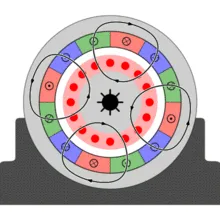Image source: https://en.wikipedia.org/wiki/Induction_motor

### DC vs AC

So, when do we use each type? The biggest advantage of AC induction motors is that they are simpler to make. The only moving part is the rotor, which makes them low-cost, quiet and long-lasting. DC motors of course have a commutator with brushes that wear out and are also relatively noisy because of the friction between those components. But, the speed of the induction motor depends on the frequency of the alternating current and so turns in a constant speed unless you use a variable-frequency drive. The speed in DC motors on the other hand is much easier to control, cause you just have to supply more or less voltage. DC motors can be directly driven by DC power. For AC motors we have to use an inverter that turns DC in AC.

## REFERENCES:

Mathematical equations that I had in this post where drawn using quicklatex!

### Electric fields:

Getting into Electromagnetism -> electromagnetim, electric charge, conductors, insulators, quantization

Coulomb's law with examples -> Coulomb's law, superposition principle, Coulomb constant, how to solve problems, examples

Electric fields and field lines -> Electric fields, Solving problems around Electric fields and field lines

Electric dipoles -> Electric dipole, torque, potential and field

Electric charge and field Exercises -> examples in electric charges and fields

### Electric flux:

Electric flux and Gauss's law -> Electric flux, Gauss's law

Applications of Gauss's law (part 1) -> applying Gauss's law, Gauss applications

Applications of Gauss's law (part 2) -> more Gauss applications

Electric flux exercises -> examples in electric flux and Gauss's law

### Electric potential:

Electric potential energy -> explanation of work-energy, electric potential energy

Calculating electric potentials -> more stuff about potential energy, potential, calculating potentials

Millikan's Oil Drop Experiment -> Millikan's experiment, electronvolt

Cathode ray tubes explained using electric potential -> cathode ray tube explanation

Electric potential exercises (part 1) -> applications of potential

Electric potential exercises (part 2) -> applications of potential gradient, advanced examples

### Capacitance:

Capacitors (Condensers) and Capacitance -> Capacitors, capacitance, calculating capacitance

How to solve problems around Capacitors -> combination, solving problems, simple example

Electric field energy and density -> Electric field energy, energy density

Dielectric materials -> Dielectrics, dielectric constant, permittivity and strength, how to solve problems

Electric capacitance exercises -> examples in capacitance, energy density and dielectrics

### Current, resistance and EMF:

Electric current -> Electric current, current density

Electrical resistivity and conductivity -> Electrical resistivity, conductivity, thermal coefficient of resistivity, hyperconductivity

Electric resistance -> Resistance, temperature, resistors

Electromotive Force (EMF) and Internal resistance -> Electromotive force, internal resistance

Power and Wattage of Electronic Circuits -> Power in general, power/wattage of electronic circuits

Electric current, resistance and emf exercises -> exampes in all those topics

### Direct current (DC) circuits:

Resistor Combinations -> Resistor combinations, how to solve problems

Kirchhoff's laws with applications -> Kirchhoff's laws, how to solve problems, applications

Electrical measuring instruments -> what are they?, types list, getting into some of them, an application

Electronic circuits with resistors and capacitors (R-C) -> R-C Circuit, charging, time constant, discharging, how to apply

RC circuit exercises -> examples in Kirchhoff, charging, discharging capacitor with/without internal resistance

### Magnetic field and forces:

Magnetic fields -> Magnetism, Magnetic field

Magnetic field lines and Gauss's law of Magnetism -> magnetic field lines, mono- and dipoles, Flux, Gauss's law of magnetism

The motion of charged particles inside of a magnetic field -> straight-line, spiral and helical particle motion

Applications of charged particle motion -> CERN, Cyclotrons, Synchrotrons, Cavity Magetron, Mass Spectrometry and Magnetic lens

Magnetic force applied on Current-Carrying Conductors -> magnetic force on current-carrying conductors/wires, proofs

Magnetic force and torque applied on current loops (circuits) -> magnetic force on current loops, magnetic moment and torque

And this is actually it for today's post and I hope that you enjoyed it!

Next time we will get into in-depth Exercises/Examples in everything that we covered in Magnetic field's and forces till now!

Bye!

H2
H3
H4
3 columns
2 columns
1 column